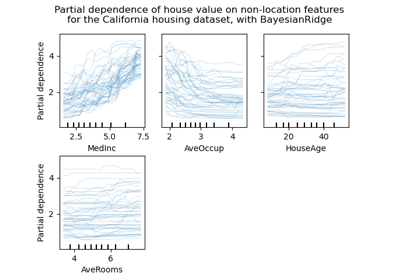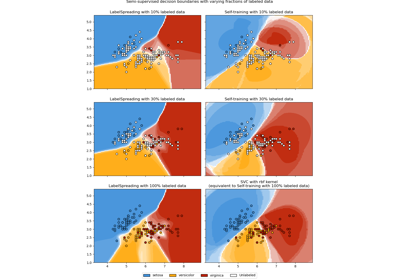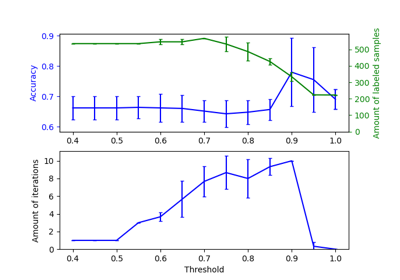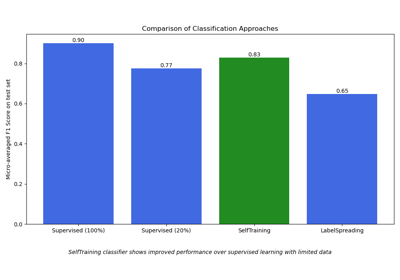# sklearn.semi_supervised.SelfTrainingClassifier¶

class sklearn.semi_supervised.SelfTrainingClassifier(base_estimator, threshold=0.75, criterion='threshold', k_best=10, max_iter=10, verbose=False)[source]

Self-training classifier.

This metaestimator allows a given supervised classifier to function as a semi-supervised classifier, allowing it to learn from unlabeled data. It does this by iteratively predicting pseudo-labels for the unlabeled data and adding them to the training set.

The classifier will continue iterating until either max_iter is reached, or no pseudo-labels were added to the training set in the previous iteration.

Read more in the User Guide.

Parameters:
base_estimatorestimator object

An estimator object implementing fit and predict_proba. Invoking the fit method will fit a clone of the passed estimator, which will be stored in the base_estimator_ attribute.

thresholdfloat, default=0.75

The decision threshold for use with criterion='threshold'. Should be in [0, 1). When using the 'threshold' criterion, a well calibrated classifier should be used.

criterion{‘threshold’, ‘k_best’}, default=’threshold’

The selection criterion used to select which labels to add to the training set. If 'threshold', pseudo-labels with prediction probabilities above threshold are added to the dataset. If 'k_best', the k_best pseudo-labels with highest prediction probabilities are added to the dataset. When using the ‘threshold’ criterion, a well calibrated classifier should be used.

k_bestint, default=10

The amount of samples to add in each iteration. Only used when criterion='k_best'.

max_iterint or None, default=10

Maximum number of iterations allowed. Should be greater than or equal to 0. If it is None, the classifier will continue to predict labels until no new pseudo-labels are added, or all unlabeled samples have been labeled.

verbosebool, default=False

Enable verbose output.

Attributes:
base_estimator_estimator object

The fitted estimator.

classes_ndarray or list of ndarray of shape (n_classes,)

Class labels for each output. (Taken from the trained base_estimator_).

transduction_ndarray of shape (n_samples,)

The labels used for the final fit of the classifier, including pseudo-labels added during fit.

labeled_iter_ndarray of shape (n_samples,)

The iteration in which each sample was labeled. When a sample has iteration 0, the sample was already labeled in the original dataset. When a sample has iteration -1, the sample was not labeled in any iteration.

n_features_in_int

Number of features seen during fit.

New in version 0.24.

feature_names_in_ndarray of shape (n_features_in_,)

Names of features seen during fit. Defined only when X has feature names that are all strings.

New in version 1.0.

n_iter_int

The number of rounds of self-training, that is the number of times the base estimator is fitted on relabeled variants of the training set.

termination_condition_{‘max_iter’, ‘no_change’, ‘all_labeled’}

The reason that fitting was stopped.

• 'max_iter': n_iter_ reached max_iter.

• 'no_change': no new labels were predicted.

• 'all_labeled': all unlabeled samples were labeled before max_iter was reached.

LabelPropagation

Label propagation classifier.

LabelSpreading

Label spreading model for semi-supervised learning.

References

David Yarowsky. 1995. Unsupervised word sense disambiguation rivaling supervised methods. In Proceedings of the 33rd annual meeting on Association for Computational Linguistics (ACL ‘95). Association for Computational Linguistics, Stroudsburg, PA, USA, 189-196.

Examples

>>> import numpy as np
>>> from sklearn import datasets
>>> from sklearn.semi_supervised import SelfTrainingClassifier
>>> from sklearn.svm import SVC
>>> rng = np.random.RandomState(42)
>>> random_unlabeled_points = rng.rand(iris.target.shape) < 0.3
>>> iris.target[random_unlabeled_points] = -1
>>> svc = SVC(probability=True, gamma="auto")
>>> self_training_model = SelfTrainingClassifier(svc)
>>> self_training_model.fit(iris.data, iris.target)
SelfTrainingClassifier(...)


Methods

 Call decision function of the base_estimator. fit(X, y) Fit self-training classifier using X, y as training data. Raise NotImplementedError. get_params([deep]) Get parameters for this estimator. Predict the classes of X. Predict log probability for each possible outcome. Predict probability for each possible outcome. score(X, y) Call score on the base_estimator. set_params(**params) Set the parameters of this estimator.
decision_function(X)[source]

Call decision function of the base_estimator.

Parameters:
X{array-like, sparse matrix} of shape (n_samples, n_features)

Array representing the data.

Returns:
yndarray of shape (n_samples, n_features)

Result of the decision function of the base_estimator.

fit(X, y)[source]

Fit self-training classifier using X, y as training data.

Parameters:
X{array-like, sparse matrix} of shape (n_samples, n_features)

Array representing the data.

y{array-like, sparse matrix} of shape (n_samples,)

Array representing the labels. Unlabeled samples should have the label -1.

Returns:
selfobject

Fitted estimator.

Raise NotImplementedError.

This estimator does not support metadata routing yet.

get_params(deep=True)[source]

Get parameters for this estimator.

Parameters:
deepbool, default=True

If True, will return the parameters for this estimator and contained subobjects that are estimators.

Returns:
paramsdict

Parameter names mapped to their values.

predict(X)[source]

Predict the classes of X.

Parameters:
X{array-like, sparse matrix} of shape (n_samples, n_features)

Array representing the data.

Returns:
yndarray of shape (n_samples,)

Array with predicted labels.

predict_log_proba(X)[source]

Predict log probability for each possible outcome.

Parameters:
X{array-like, sparse matrix} of shape (n_samples, n_features)

Array representing the data.

Returns:
yndarray of shape (n_samples, n_features)

Array with log prediction probabilities.

predict_proba(X)[source]

Predict probability for each possible outcome.

Parameters:
X{array-like, sparse matrix} of shape (n_samples, n_features)

Array representing the data.

Returns:
yndarray of shape (n_samples, n_features)

Array with prediction probabilities.

score(X, y)[source]

Call score on the base_estimator.

Parameters:
X{array-like, sparse matrix} of shape (n_samples, n_features)

Array representing the data.

yarray-like of shape (n_samples,)

Array representing the labels.

Returns:
scorefloat

Result of calling score on the base_estimator.

set_params(**params)[source]

Set the parameters of this estimator.

The method works on simple estimators as well as on nested objects (such as Pipeline). The latter have parameters of the form <component>__<parameter> so that it’s possible to update each component of a nested object.

Parameters:
**paramsdict

Estimator parameters.

Returns:
selfestimator instance

Estimator instance.

## Examples using sklearn.semi_supervised.SelfTrainingClassifier¶Release Highlights for scikit-learn 0.24

Release Highlights for scikit-learn 0.24Decision boundary of semi-supervised classifiers versus SVM on the Iris dataset

Decision boundary of semi-supervised classifiers versus SVM on the Iris datasetEffect of varying threshold for self-training

Effect of varying threshold for self-trainingSemi-supervised Classification on a Text Dataset

Semi-supervised Classification on a Text Dataset